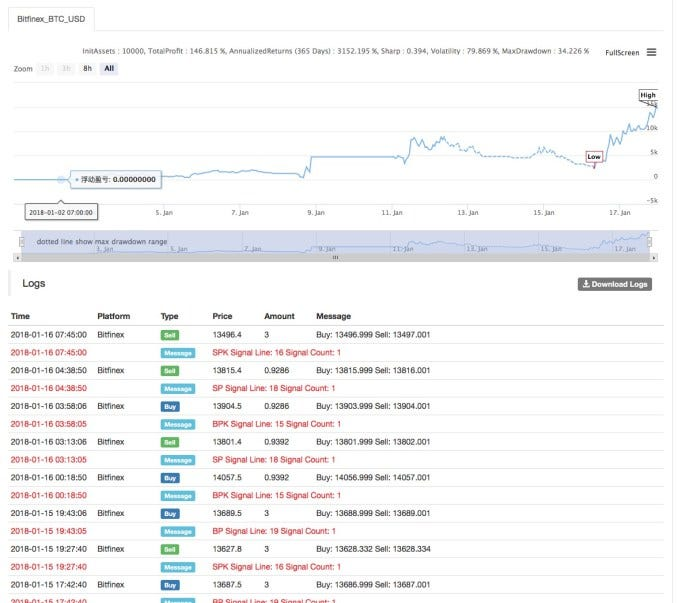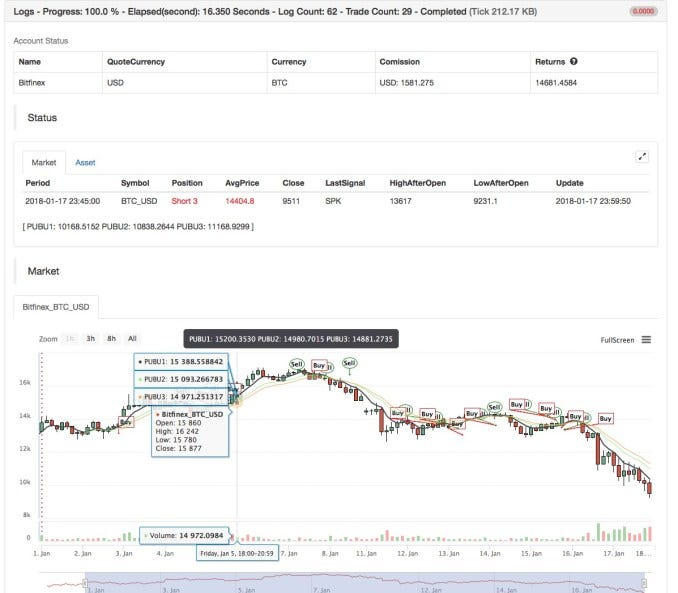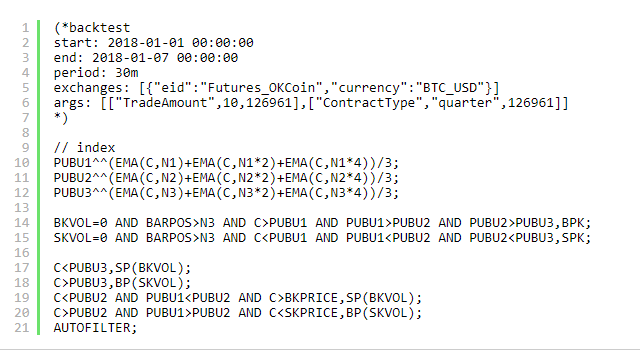#1
Strategy Name: PBX Strategy

Data cycle: 15M

Support: Commodity Futures, cryptocurrencyMain chart:
PBX1，formula：PUBU1^^(EMA(C,N1)+EMA(C,N12)+EMA(C,N14))/3;
PBX2，formula：PUBU2^^(EMA(C,N2)+EMA(C,N22)+EMA(C,N24))/3;
PBX3，formula：PUBU3^^(EMA(C,N3)+EMA(C,N32)+EMA(C,N34))/3;-------------------------------------------
Source Code: https://www.fmz.com/strategy/128420
0 x